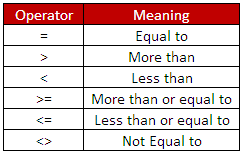# Excel VBA : IF THEN ELSE STATEMENT

This tutorial guides you through the basics of conditional and logical operators and shows you how to use IF THEN ELSE statement in excel VBA.

Conditional OperatorsLogical OperatorsIF THEN Statement
The syntax for IF THEN statement is as follows :
If (condition1) Then
' code if condition1 is TRUE
End If
Example
If student's grade is greater than or equal to 60, he/she will be considered as having passed the examination.

Let's create a user defined function (UDF) that accomplishes the above task -
Function examresult(score As Single) As String
If score >= 60 Then
examresult = "PASS"
End If
End Function

How this works:
1. The If statement checks the condition "score >= 60"
2. The "score >= 60" condition will return either TRUE or FALSE.
3. If the "score >= 60" condition evaluates to TRUE then examresult function will return PASS.
4. If the "score >= 60" condition evaluates to FALSE then examresult function will return blank because you have not specified ELSE statement (By default, it returns 0 for function with a numeric type and blank for function with a string type)
5. End if is necessary to complete an If statement.

IF THEN ELSE Statement
The syntax for IF THEN ELSE statement is as follows :
If Condition1 Then
'code if condition1 is True
Else
'code if condition1 is False
End If
Example
If student's grade is greater than or equal to 60, he/she will be considered as having passed the examination. Else he/she will be considered as having failed the examination.

Let's create a user defined function (UDF) that accomplishes the above task -
Function examresult(score As Single) As String
If score >= 60 Then
examresult = "PASS"
Else
examresult = "FAIL"
End If
End Function

How this works:
1. The If statement checks the condition "score >= 60"
2. The "score >= 60" condition will return either TRUE or FALSE.
3. If the "score >= 60" condition evaluates to TRUE then examresult function will return PASS.
4. If the "score >= 60" condition evaluates to FALSE then examresult function will return FAIL
5. End if is necessary to complete an If statement.

IF THEN ELSEIF ELSE Statement
The syntax for IF THEN ELSEIF ELSE statement is as follows :
If Condition1 Then
'code if condition1 is True
ElseIf condition2 Then
'code if condition1 is FALSE AND condition2 is TRUE
Else
'code if Condition1 is FALSE AND Condition2 is FALSE
End If
Example
If student's score is greater than or equal to 75, he/she will get A grade.
If student's score is less than 75 but greater than 50, he/she will get B grade.
If student's score is less than or equal to 50, he/she will get C grade.

Let's create a user defined function (UDF) that accomplishes the above task -
Function grade(score As Single) As String
If score >= 75 Then
ElseIf score > 50 Then
Else
End If
End Function
Another way of writing the above program -
Function grade1(score As Single) As String
If score >= 0 and score <= 50 Then
ElseIf score > 50 and score < 75 Then
Else
End If
End Function
How to use
1. Open an Excel Workbook
2. Press Alt+F11 to open VBA Editor
3. Go to Insert Menu >> Module
4. Copy the above code and Paste it into module
5. Save the file as Macro Enabled Workbook (xlsm) or Excel 97-2003 Workbook (xls)
6. Insert user defined function by typing the function i.e. =grade(cell_ref)
Related Posts
Share